# NCERT Solutions Class 12 Mathematics Chapter 12 Linear Programming

## Chapter 12 Linear Programming Class 12 Mathematics NCERT Solutions

Class 12 Mathematics students should refer to the following NCERT questions with answers for Chapter 12 Linear Programming in Class 12. These NCERT Solutions with answers for Class 12 Mathematics will come in exams and help you to score good marks

### Chapter 12 Linear Programming NCERT Solutions Class 12 Mathematics

Exercise 12.1

Question1.  Maximize Z = 3x + 4y subject to the constraints: x + y ≤ 4, x ≥ 0, y ≥ 0.

The feasible region determined by the constraints, x y ≤ 4, x ≥ 0, y ≥ 0, is as follows.The corner points of the feasible region are O (0, 0), A (4, 0), and B (0, 4). The values of Z at these points are as follows.Therefore, the maximum value of Z is 16 at the point B (0, 4).

Question2.  Minimise Z = – 3x + 4 y subject to x + 2y ≤ 8, 3x + 2y ≤ 12, x ≥ 0, y ≥ 0.

The feasible region determined by the system of constraints,x + 2y ≤ 8, 3x + 2y ≤ 12, x ≥ 0, and y ≥ 0, is as follows.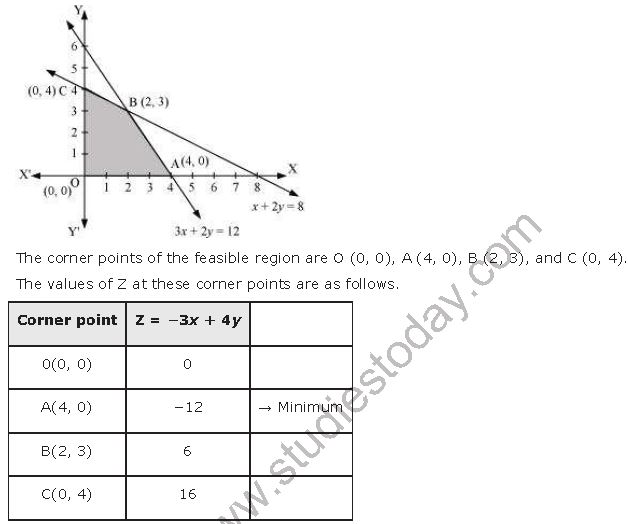Therefore, the minimum value of Z is −12 at the point (4, 0).

Question3. Maximise Z = 5x + 3y subject to 3x + 5y ≤ 15, 5x + 2y ≤ 10, x ≥ 0, y ≥ 0

The feasible region determined by the system of constraints, 3x + 5y ≤ 15,

5x + 2y ≤ 10, x ≥ 0, and y ≥ 0, are as follows.Therefore, the maximum value of Z is 235/19 at the point (20/19, 45/19).

Question4. Minimise Z = 3x + 5y such that x + 3y ≥ 3, x + y ≥ 2, x, y ≥ 0.

The feasible region determined by the system of constraints, x + 3y ≥ 3, x + y ≥ 2, and xy ≥ 0, is as follows.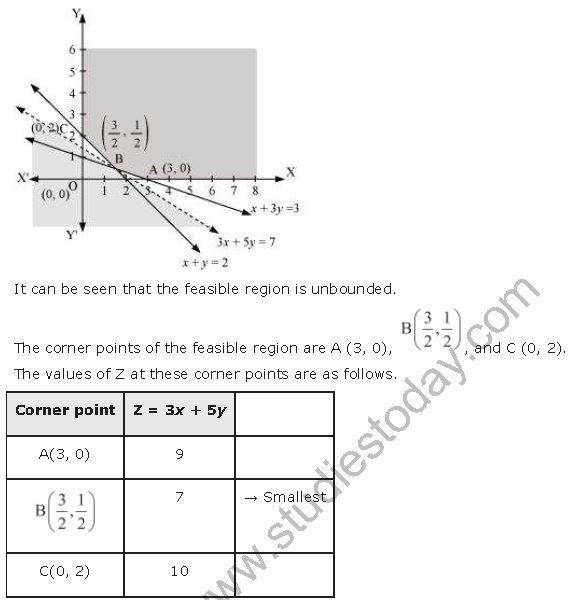As the feasible region is unbounded, therefore, 7 may or may not be the minimum value of Z.

For this, we draw the graph of the inequality, 3x + 5y < 7, and check whether the resulting half plane has points in common with the feasible region or not.

It can be seen that the feasible region has no common point with 3x + 5y < 7 Therefore, the minimum value of Z is 7 at (3/2,1/2).

Question5.  Maximise Z = 3x + 2y subject to x + 2y ≤ 10, 3x + y ≤ 15, x, y ≥ 0.

The feasible region determined by the constraints, x + 2y ≤ 10, 3x + ≤ 15, x ≥ 0, and y ≥ 0, is as follows.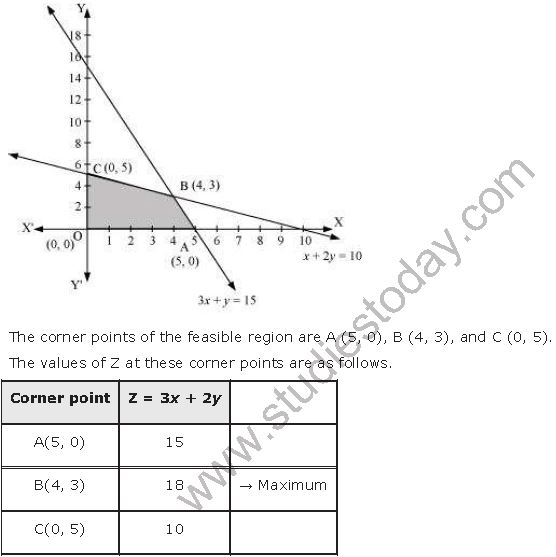Therefore, the maximum value of Z is 18 at the point (4, 3).

Question6.  Minimise Z = x + 2y subject to 2x + y ≥ 3, x + 2y ≥ 6, x, y ≥ 0.

The feasible region determined by the constraints, 2x + y ≥ 3, x + 2y ≥ 6, x ≥ 0, and y ≥ 0, is as follows.It can be seen that the value of Z at points A and B is same. If we take any other point such as (2, 2) on line x + 2y = 6, then Z = 6

Thus, the minimum value of Z occurs for more than 2 points.

Therefore, the value of Z is minimum at every point on the line, x + 2y = 6

Question7.  Minimise and Maximise Z = 5x + 10 y subject to x + 2y ≤ 120, x + y ≥ 60, x – 2y ≥ 0, x, y ≥ 0.

The feasible region determined by the constraints, x + 2y ≤ 120, x + y ≥ 60, x − 2y ≥ 0, x ≥ 0, and y ≥ 0, is as follows.The minimum value of Z is 300 at (60, 0) and the maximum value of Z is 600 at all the points on the line segment joining (120, 0) and (60, 30).

Question8.  Minimise and Maximise Z = x + 2y subject to x + 2y ≥ 100, 2x – y ≤ 0, 2x + y ≤ 200; x, y ≥ 0.

The feasible region determined by the constraints, x + 2y ≥ 100, 2x − y ≤ 0, 2x + ≤ 200, x ≥ 0, and y ≥ 0, is as follows.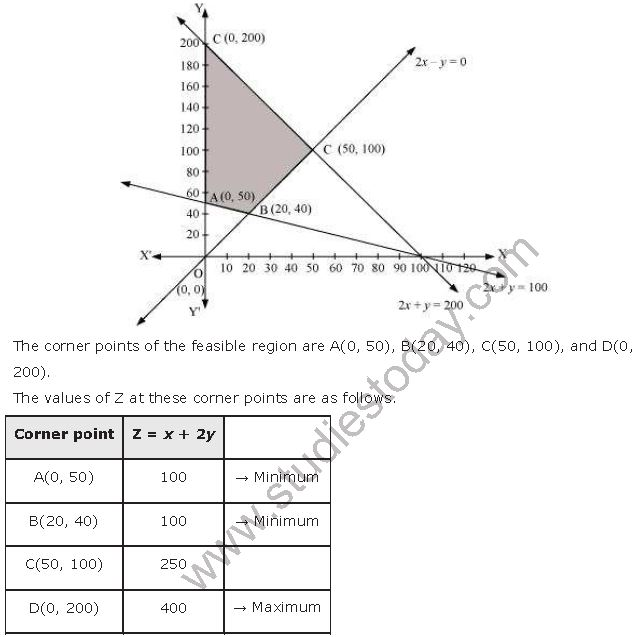The maximum value of Z is 400 at (0, 200) and the minimum value of Z is 100 at all the points on the line segment joining the points (0, 50) and (20, 40).

Question9.  Maximise Z = – x + 2y, subject to the constraints x ≥ 3, x + y ≥ 5, x + 2y ≥ 6, y ≥ 0.

The feasible region determined by the constraints, x ≥ 3, x + y ≥ 5, x + 2y ≥ 6 and y ≥ 0 is as follows.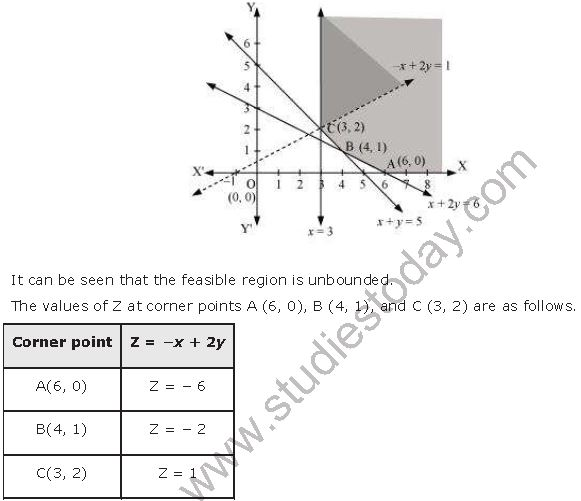As the feasible region is unbounded, therefore, Z = 1 may or may not be the maximum value.

For this, we graph the inequality, −x + 2y > 1, and check whether the resulting half plane has points in common with the feasible region or not.

The resulting feasible region has points in common with the feasible region.

Therefore, Z = 1 is not the maximum value. Z has no maximum value.

Question10.  Maximise Z = x + y, subject to x – y ≤ –1, –x + y ≤ 0, x, y ≥ 0.

The region determined by the constraints, x – y ≤ –1, –x + y ≤ 0, x, y ≥ 0 is as follows.Exercise 12.2

Question1. Reshma wishes to mix two types of food P and Q in such a way that the vitamin contents of the mixture contain at least 8 units of vitamin A and 11 units of vitamin B. Food P costs Rs. 60/kg and Food Q costs Rs. 80/kg. Food P contains 3 units/kg of vitamin A and 5 units/kg of vitamin B while Food Q contains 4 units/kg of vitamin A and 2 units/kg of vitamin B. Determine the minimum cost of the mixture.

Let the mixture contain x kg of food P and y kg of food Q. Therefore,

x ≥ 0 and y ≥ 0

The given information can be compiled in a table as follows.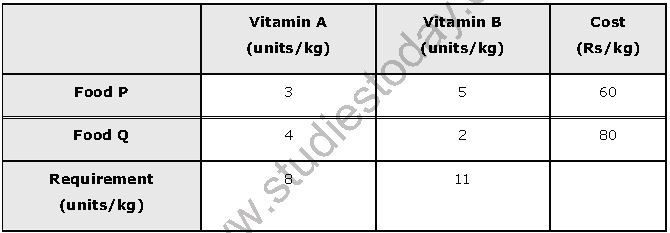The mixture must contain at least 8 units of vitamin A and 11 units of vitamin B. Therefore, the constraints are

3x + 4y ≥ 8

5x + 2y ≥ 11

Total cost, Z, of purchasing food is, Z = 60x + 80y

The mathematical formulation of the given problem is

Minimise Z = 60x + 80y … (1)

subject to the constraints,

3x + 4y ≥ 8 … (2)

5x + 2y ≥ 11 … (3)

xy ≥ 0 … (4)

The feasible region determined by the system of constraints is as follows.As the feasible region is unbounded, therefore, 160 may or may not be the minimum value of Z.

For this, we graph the inequality, 60x + 80y < 160 or 3x + 4y < 8, and check whether the resulting half plane has points in common with the feasible region or not.

It can be seen that the feasible region has no common point with 3x + 4y < 8

Therefore, the minimum cost of the mixture will be Rs 160 at the line segment joining the points (8/3,0) and (2,1/2).

Question2. One kind of cake requires 200g of flour and 25 g of fat and another kind of cake requires 100 g of flour and 50 g of fat. Find the maximum number of cake which can be made from 5 kg of flour and 1 kg of fat assuming that there is no shortage of the other ingredients used in making the cakes.

Let there be x cakes of first kind and y cakes of second kind. Therefore,

x ≥ 0 and y ≥ 0

The given information can be complied in a table as follows.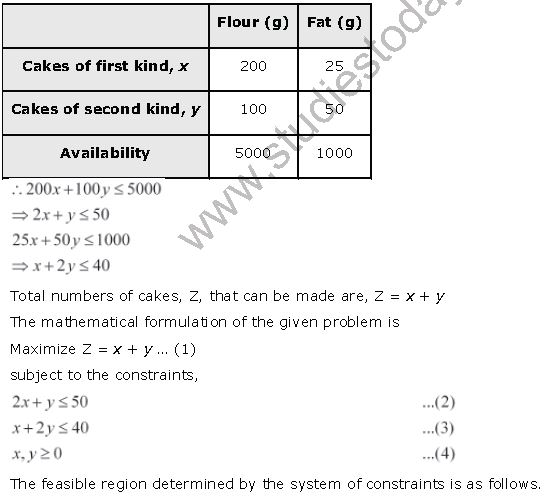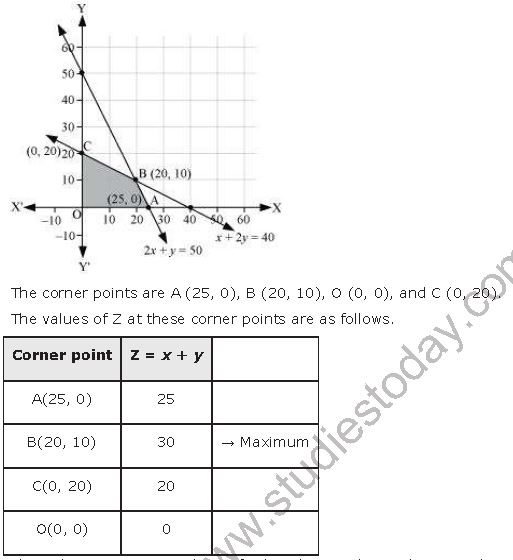Thus, the maximum numbers of cakes that can be made are 30 (20 of one kind and 10 of the other kind).

Question3. A factory makes tennis rackets and cricket bats. A tennis racket takes 1.5 hours of machine time and 3 hours of craftman’s time in its making while a cricket bat takes 3 hours of machine time and 1 hour of craftman’s time. In a day, the factory has the availability of not more than 42 hours of machine time and 24 hours of craftman’s time.

(i) What number of rackets and bats must be made if the factory is to work at full capacity?

(ii) If the profit on a racket and on a bat is Rs. 20 and Rs. 10 respectively, find maximum profit of the factory when it works at full capacity.

(i) Let the number of rackets and the number of bats to be made be x and y respectively.

The machine time is not available for more than 42 hours.

∴ 1.5x + 3y ≤ 42 ....(1)

The craftsman’s time is not available for more than 24 hours.

∴ 3x + y ≤ 24              ......(2)

The factory is to work at full capacity. Therefore,

1.5x + 3y = 42

3x + y = 24

On solving these equations, we obtain

x = 4 and y = 12

Thus, 4 rackets and 12 bats must be made.

(i) The given information can be complied in a table as follows.∴ 1.5x + 3y ≤ 42

3x + y ≤ 24

xy ≥ 0

The profit on a racket is Rs 20 and on a bat is Rs 10.

∴ Z = 20x + 10y

The mathematical formulation of the given problem is

Maximize Z = 20x + 10y … (1)

subject to the constraints,

1.5x + 3y ≤ 42 … (2)

3x + y ≤ 24 … (3)

xy ≥ 0 … (4)

The feasible region determined by the system of constraints is as follows.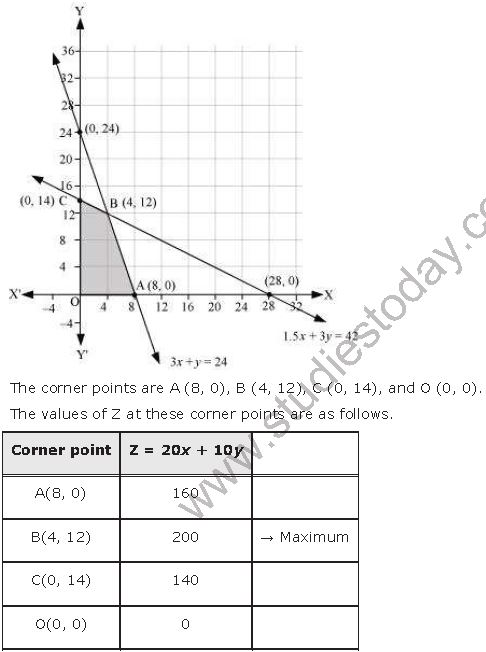Thus, the maximum profit of the factory when it works to its full capacity is Rs 200.

Question4. A manufacturer produces nuts and bolts. It takes 1 hour of work on machine A and 3 hours on machine B to produce a package of nuts. It takes 3 hours on machine A and 1 hour on machine B to produce a package of bolts. He earns a profit of Rs. 17.50 per package on nuts and Rs. 7.00 per package on bolts. How many packages of each should be produced each day so as to maximize his profit, if he operates his machines for at the most 12 hours a day?

Let the manufacturer produce x packages of nuts and y packages of bolts. Therefore,

x ≥ 0 and y ≥ 0

The given information can be compiled in a table as follows.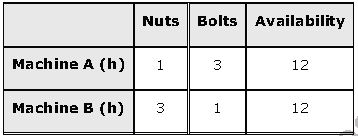The profit on a package of nuts is Rs 17.50 and on a package of bolts is Rs 7. Therefore, the constraints are

x + 3y ≤ 12 … (2)

3x + y ≤ 12 … (3

Total profit, Z = 17.5x + 7y

The mathematical formulation of the given problem is

Maximise Z = 17.5x + 7y … (1)

subject to the constraints,

x + 3y ≤ 12 … (2)

3x + y ≤ 12 … (3)

xy ≥ 0 … (4)

The feasible region determined by the system of constraints is as follows.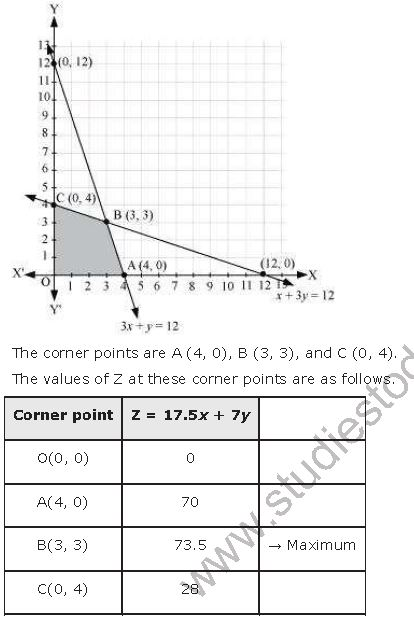The maximum value of Z is Rs 73.50 at (3, 3).

Thus, 3 packages of nuts and 3 packages of bolts should be produced each day to get the maximum profit of Rs 73.50.

Question5. A factory manufacturers two types of screws, A and B. Each type of screw requires the use of two machines, an automatic and a hand operated. It takes 4 minutes on the automatic and 6 minutes on hand operated machines to manufacture a package of screw A, while it takes 6 minutes on automatic and 3 minutes on hand operated machines to manufacture a package of screws B. Each machine is available for at the most 4 hours on any day. The manufacturer can sell a package of screws A at a profit of Rs. 7 and screws B at a profit of Rs. 10. Assuming that he can sell all the screws he manufactures, how many packages of each type should the factory owner produce a day in order to maximize his profit? Determine the maximum profit.

Let the factory manufacture x screws of type A and y screws of type B on each day. Therefore,

x ≥ 0 and y ≥ 0

The given information can be compiled in a table as follows.The profit on a package of screws A is Rs 7 and on the package of screws B is Rs 10. Therefore, the constraints are

4x + 6y ≤ 240

6x + 3y ≤ 240

Total profit, Z = 7x + 10y

The mathematical formulation of the given problem is

Maximize Z = 7x + 10y … (1)

subject to the constraints,

4x + 6y ≤ 240      ....(2)

6x + 3y ≤ 240     .....(3)

xy ≥ 0 … (4)

The feasible region determined by the system of constraints isThe maximum value of Z is 410 at (30, 20).

Thus, the factory should produce 30 packages of screws A and 20 packages of screws B to get the maximum profit of Rs 410.

Question6. A cottage industry manufactures pedestal lamps and wooden shades, each requiring the use of a grinding/cutting machine and a sprayer. It takes 2 hours on grinding/cutting machine and 3 hours on the sprayer to manufacture a pedestal lamp. It takes 1 hour on the grinding/cutting machine and 2 hours on the sprayer to manufacture a shade. On any day, the sprayer is available for at the most 20 hours and the grinding/cutting machine for at the most 12 hours. The profit from the sale of a lamp is Rs.5 and that from a shade is Rs.3. Assuming that the manufacturer can sell all the lamps and shades that he produces, how should he schedule his daily production in order to maximize his profit?

Let the cottage industry manufacture x pedestal lamps and y wooden shades. Therefore,

x ≥ 0 and y ≥ 0

The given information can be compiled in a table as follows.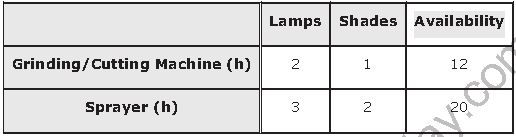The profit on a lamp is Rs 5 and on the shades is Rs 3. Therefore, the constraints are

2x + y ≤ 12

3x + 2y ≤ 20

Total profit, Z = 5x + 3y

The mathematical formulation of the given problem is

Maximize Z = 5x + 3y … (1)

subject to the constraints,

2x + y ≤ 12 … (2)

3x + 2y ≤ 20 … (3)

xy ≥ 0 … (4)

The feasible region determined by the system of constraints is as follows.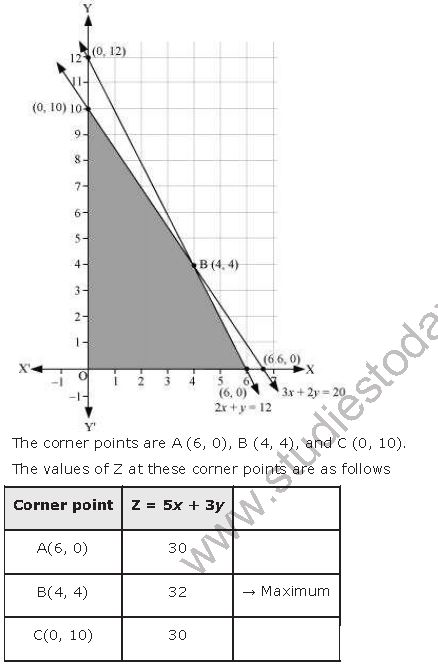The maximum value of Z is 32 at (4, 4).

Thus, the manufacturer should produce 4 pedestal lamps and 4 wooden shades to maximize his profits.

Question7. A company manufactures two types of novelty souvenirs made of plywood. Souvenirs of type A requires 5 minutes each for cutting and 10 minutes each for assembling. Souvenirs of type B require 8 minutes each for cutting and 8 minutes each for assembling. There are 3 hours 20 minutes available for cutting and 4 hours for assembling. The profit is Rs.5 each for type A and Rs.6 each for type B souvenirs. How many souvenirs of each type should the company manufacture in order to maximize the profit?

Let the company manufacture x souvenirs of type A and y souvenirs of type B. Therefore,

x ≥ 0 and y ≥ 0

The given information can be complied in a table as follows.The profit on type A souvenirs is Rs 5 and on type B souvenirs is Rs 6. Therefore, the constraints are

5x + 8y ≤ 200

10x + 8y ≤ 240 i.e.,5x + 4y ≤ 120

Total profit, Z = 5x + 6y

The mathematical formulation of the given problem is

Maximize Z = 5x + 6y … (1)

subject to the constraints,

5x + 8y ≤ 200 … (2)

5x + 4y ≤ 120 … (3)

xy ≥ 0 … (4)

The feasible region determined by the system of constraints is as follows.The maximum value of Z is 200 at (8, 20).

Thus, 8 souvenirs of type A and 20 souvenirs of type B should be produced each day to get the maximum profit of Rs 160.

Question8. A merchant plans to sell two types of personal computers – a desktop model and a portable model that will cost Rs. 25000 and Rs. 40000 respectively. He estimates that the total monthly demand of computers will not exceed 250 units. Determine the number of units of each type of computers which the merchant should stock to get maximum profit if he does not want to invest more than Rs, 70 lakhs and if his profit on the desktop model is Rs. 4500 and on portable model is Rs. 5000.

Let the merchant stock x desktop models and y portable models. Therefore,

x ≥ 0 and y ≥ 0

The cost of a desktop model is Rs 25000 and of a portable model is Rs 4000. However, the merchant can invest a maximum of Rs 70 lakhs.

∴ 25000x + 40000y ≤ 7000000

5x +8y ≤ 1400

The monthly demand of computers will not exceed 250 units.

∴ x + y ≤ 250

The profit on a desktop model is Rs 4500 and the profit on a portable model is Rs 5000.

Total profit, Z = 4500x + 5000y

Thus, the mathematical formulation of the given problem is

Maximum Z = 4500x + 5000y              .....(1)

subject to the constraints,

5x +8y ≤ 1400      ....(2)

∴ x + y ≤ 250       .....(3)

x, y ≥ 01400        ......(4)

The feasible region determined by the system of constraints is as follows.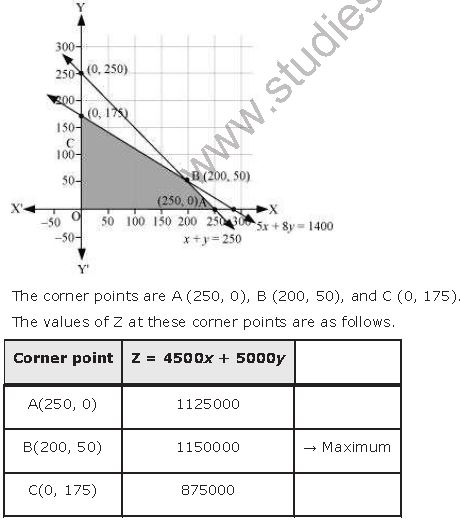The maximum value of Z is 1150000 at (200, 50).

Thus, the merchant should stock 200 desktop models and 50 portable models to get the maximum profit of Rs 1150000.

Question9. A diet is to contain at least 80 units of vitamin A and 100 units of minerals. Two foods F1 and F2 are available. Food F1 costs Rs. 4 per unit food and F2 costs Rs. 6 per unit. One unit of food F1 contains 3 units of vitamin A and 4 units of minerals. One unit of food F2 contains 6 units of vitamin A and 3 units of minerals. Formulate this as a linear programming problem. Find the minimum cost for diet that consists of mixture of these two foods and also meets the minimal nutritional requirements.

Let the diet contain units of food F1 and y units of food F2. Therefore,

x ≥ 0 and y ≥ 0

The given information can be complied in a table as follows.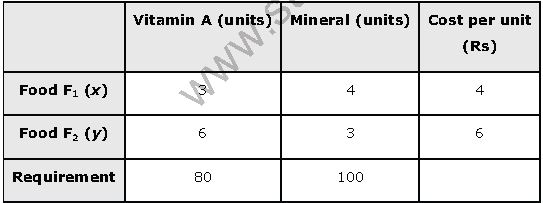The cost of food F1 is Rs 4 per unit and of Food F2 is Rs 6 per unit. Therefore, the constraints are

3x + 6y ≥ 80

4x + 3y ≥ 100

xy ≥ 0

Total cost of the diet, Z = 4x + 6y

The mathematical formulation of the given problem is

Minimise Z = 4x + 6y … (1)

subject to the constraints,

3x + 6y ≥ 80 … (2)

4x + 3y ≥ 100 … (3)

xy ≥ 0 … (4)

The feasible region determined by the constraints is as follows.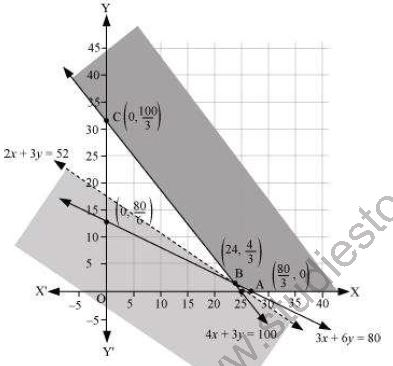As the feasible region is unbounded, therefore, 104 may or may not be the minimum value of Z.

For this, we draw a graph of the inequality, 4x + 6y < 104 or 2x + 3y < 52, and check whether the resulting half plane has points in common with the feasible region or not.

It can be seen that the feasible region has no common point with 2x + 3y < 52

Therefore, the minimum cost of the mixture will be Rs 104.

Question10. There are two types of fertilizers F1 and F2. F1 consists of 10% nitrogen and 6% phosphoric acid and F2 consists of 5% nitrogen and 10% phosphoric acid. After testing the soil conditions, a farmer finds that she needs at least 14 kg of nitrogen and 14 kg of phosphoric acid for her crop. If F1 costs Rs. 6/kg and Fcosts Rs. 5/kg, determine how much of each type of fertilizer should be used so that nutrient requirements are met at a minimum cost. What is the minimum cost?

Let the farmer buy x kg of fertilizer F1 and y kg of fertilizer F2. Therefore,

x ≥ 0 and y ≥ 0

The given information can be complied in a table as follows.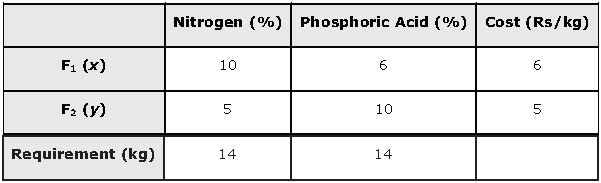F1 consists of 10% nitrogen and F2 consists of 5% nitrogen. However, the farmer requires at least 14 kg of nitrogen.

∴ 10% of + 5% of y ≥ 14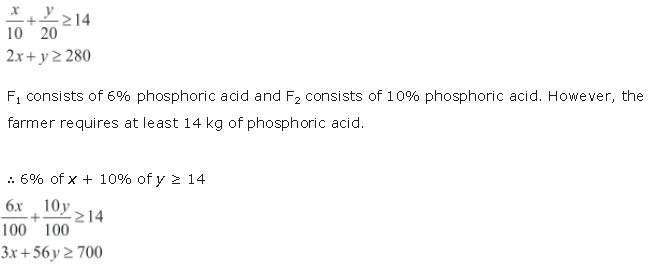Total cost of fertilizers, Z = 6x + 5y

The mathematical formulation of the given problem is

Minimize Z = 6x + 5y … (1)

subject to the constraints,

2x + y ≥ 280 … (2)

3x + 5y ≥ 700 … (3)

xy ≥ 0 … (4)

The feasible region determined by the system of constraints is as follows.As the feasible region is unbounded, therefore, 1000 may or may not be the minimum value of Z.

For this, we draw a graph of the inequality, 6x + 5y < 1000, and check whether the resulting half plane has points in common with the feasible region or not.

It can be seen that the feasible region has no common point with

6x + 5y < 1000

Therefore, 100 kg of fertiliser F1 and 80 kg of fertilizer F2 should be used to minimize the cost. The minimum cost is Rs 1000.

Question11. 2x + y ≤ 10, x + 3y ≤ 15, x, y ≥ 0 are (0, 0), (5, 0), (3, 4) and (0, 5). Let Z = px + qy, where p, q > 0. Condition on p and q so that the maximum of Z occurs at both (3, 4) and (0, 5) is
(A) p = q
(B) p = 2q
(C) p = 3q
(D) q = 3p

The maximum value of Z is unique.

It is given that the maximum value of Z occurs at two points, (3, 4) and (0, 5).

∴ Value of Z at (3, 4) = Value of Z at (0, 5)

⇒ p(3) + q(4) = p(0) + q(5)

⇒ 3p + 4= 5q

⇒ q = 3p

Hence, the correct answer is D.

Hence option (D) is correct.

Miscellaneous Solutions

Question1. Refer to Example 9. How many packets of each food should be used to maximize the amount of vitamin A in the diet? What is the maximum amount of vitamin A in the diet?

Let the diet contain and y packets of foods P and Q respectively. Therefore,
x ≥ 0 and y ≥ 0
The mathematical formulation of the given problem is as follows.
Maximize = 6+ 3y       …(1)
subject to the constraints,
4x + y ≥ 80                        ...(2)
x + 5y ≥ 115                      ...(3)
3x + 2y ≤ 150                    ...(4)
x, y ≥ 0                              ...(5)
The feasible region determined by the system of constraints is as follows.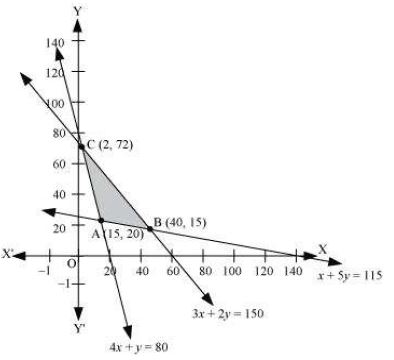The corner points of the feasible region are A (15, 20), B (40, 15), and C (2, 72).
The values of at these corner points are as follows.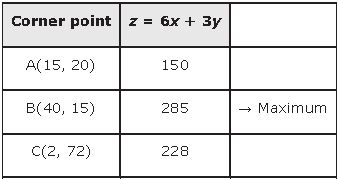Thus, the maximum value of z is 285 at (40, 15).
Therefore, to maximize the amount of vitamin A in the diet, 40 packets of food P and 15 packets of food Q should be used. The maximum amount of vitamin A in the diet is 285 units.

Question2. A farmer mixes two brands P and Q of cattle feed. Brand P, costing Rs 250 per bag contains 3 units of nutritional element A, 2.5 units of element B and 2 units of element C. Brand Q costing Rs 200 per bag contains 1.5 units of nutritional elements A, 11.25 units of element B, and 3 units of element C. The minimum requirements of nutrients A, B and C are 18 units, 45 units and 24 units respectively. Determine the number of bags of each brand which should be mixed in order to produce a mixture having a minimum cost per bag? What is the minimum cost of the mixture per bag?

Let the farmer mix x bags of brand P and y bags of brand Q.
The given information can be compiled in a table as follows.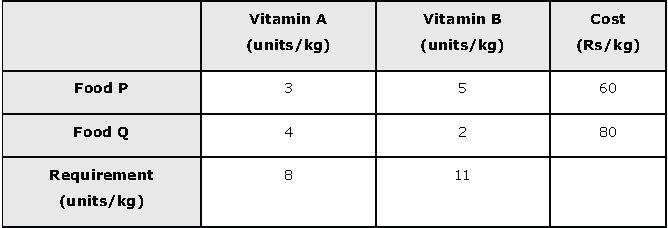The given problem can be formulated as follows.

Minimize z = 250x + 200y …(1)

subject to the constraints,

3x + 1.5y ≥ 18 …(2)

2.5x + 11.25y ≥ 45 ...(3)

2x + 3y ≥ 24 …(4)

x, y ≥ 0 …(5)

The feasible region determined by the system of constraints is as follows.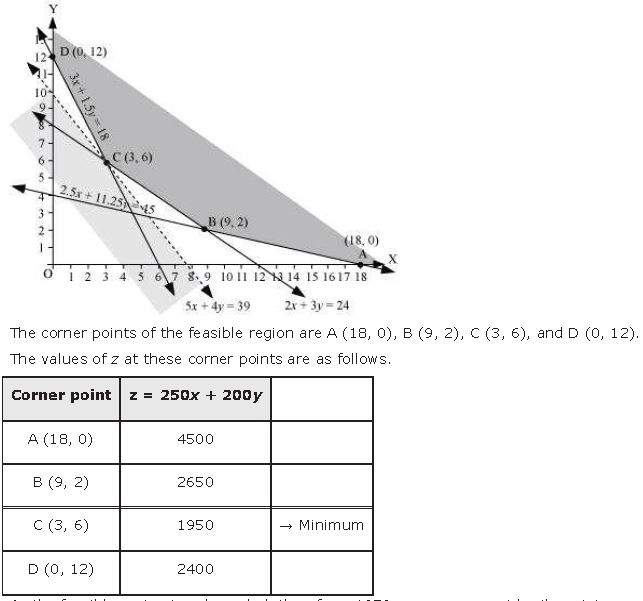As the feasible region is unbounded, therefore, 1950 may or may not be the minimum value of z.
For this, we draw a graph of the inequality, 250x + 200y < 1950 or 5x + 4y < 39, and check whether the resulting half plane has points in common with the feasible region or not.
It can be seen that the feasible region has no common point with 5x + 4y < 39
Therefore, the minimum value of z is 1950 at (3, 6).
Thus, 3 bags of brand P and 6 bags of brand Q should be used in the mixture to minimize the cost to Rs 1950.

Question3. A dietician wishes to mix together two kinds of food X and Y in such a way that the mixture contains at least 10 units of vitamin A, 12 units of vitamin B and 8 units of vitamin C. The vitamin content of one kg food is given below:One kg of food X costs Rs 16 and one kg of food Y costs Rs 20. Find the least cost of the mixture which will produce the required diet?

Let the mixture contain x kg of food X and y kg of food Y.

The mathematical formulation of the given problem is as follows.

Minimize z = 16x + 20y … (1)

subject to the constraints,

x + 2y ≥ 10 ...(2)

x + y  ≥ 6 ...(3)

3x + y ≥ 8 ...(4)

x, y ≥  0 ...(5)

The feasible region determined by the system of constraints is as follows.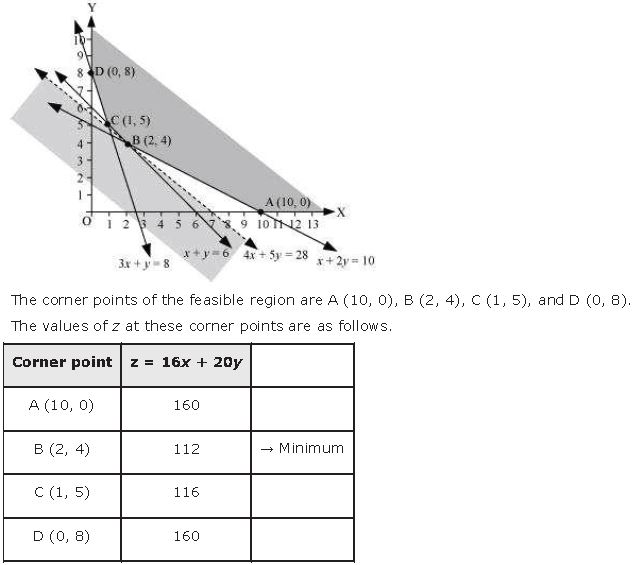As the feasible region is unbounded, therefore, 112 may or may not be the minimum value of z.
For this, we draw a graph of the inequality, 16x + 20y < 112 or 4x + 5y < 28, and check whether the resulting half plane has points in common with the feasible region or not.
It can be seen that the feasible region has no common point with 4x + 5y < 28
Therefore, the minimum value of z is 112 at (2, 4).
Thus, the mixture should contain 2 kg of food X and 4 kg of food Y. The minimum cost of the mixture is Rs 112.

Question4. A manufacturer makes two types of toys A and B. Three machines are needed for this purpose and the time (in minutes) required for each toy on the machines is given below: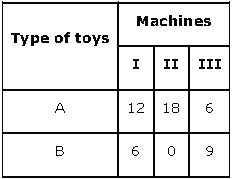Each machine is available for a maximum of 6 hours per day. If the profit on each toy of type A is Rs 7.50 and that on each toy of type B is Rs 5, show that 15 toys of type A and 30 of type B should be manufactured in a day to get maximum profit.

Let x and y toys of type A and type B respectively be manufactured in a day.

The given problem can be formulated as follows.

Maximize z = 7.5x + 5y  …(1)

subject to the constraints,

2x + y ≤ 60 …(2)

x ≤ 20 …(3)

2x + 3y ≤ 120 …(4)

x, y ≥ 0 …(5)

The feasible region determined by the constraints is as follows.The maximum value of z is 262.5 at (15, 30).
Thus, the manufacturer should manufacture 15 toys of type A and 30 toys of type B to maximize the profit.

Question5. An aeroplane can carry a maximum of 200 passengers. A profit of Rs 1000 is made on each executive class ticket and a profit of Rs 600 is made on each economy class ticket. The airline reserves at least 20 seats for executive class. However, at least 4 times as many passengers prefer to travel by economy class than by the executive class. Determine how many tickets of each type must be sold in order to maximize the profit for the airline. What is the maximum profit?

Let the airline sell tickets of executive class and y tickets of economy class.

The mathematical formulation of the given problem is as follows.
Maximize z = 1000+ 600y …(1)

subject to the constraints,

x + y ≤ 200 …(2)

x ≥ 20 …(3)

y – 4x ≥ 0 …(4)

x, y ≥ 0 …(5)

The feasible region determined by the constraints is as follows.The maximum value of z is 136000 at (40, 160).
Thus, 40 tickets of executive class and 160 tickets of economy class should be sold to maximize the profit and the maximum profit is Rs 136000.

Question6. Two godowns A and B have grain capacity of 100 quintals and 50 quintals respectively. They supply to 3 ration shops, D, E and F whose requirements are 60, 50 and 40 quintals respectively. The cost of transportation per quintal from the godowns to the shops are given in the following table: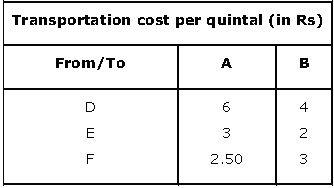How should the supplies be transported in order that the transportation cost is minimum? What is the minimum cost?

Let godown A supply x and y quintals of grain to the shops D and E respectively. Then, (100 − x − y) will be supplied to shop F.

The requirement at shop D is 60 quintals since x quintals are transported from godown A. Therefore, the remaining (60 −x) quintals will be transported from godown B.

Similarly, (50 − y) quintals and 40 − (100 − x − y) = (x + y − 60) quintals will be transported from godown B to shop E and F respectively.

The given problem can be represented diagrammatically as follows.x  ≥ 0, y ≥ 0, and  100 - x - y ≥ 0

⇒ x ≥ 0, y≥ 0, and x + y ≤ 100

60 - x ≥ 0, 50 - y ≥ 0, and x + y - 60 ≥ 0

⇒ x ≤ 60, y ≤ 50, and  x + y ≥ 60

Total transportation cost z is given by,

z = 6x + 3y +2.5(100 - x - y) + 4(60 - x) + 2(50 - y) + 3(x + y - 60)

= 6x + 3y + 250 - 2.5x - 2.5y + 240 - 4x + 100 - 2y + 3x + 3y - 180

= 2.5x + 1.5y + 410

The given problem can be formulated as

Minimize z = 2.5x + 1.5y + 410 …(1)

subject to the constraints,

x + y ≤ 100 ...(2)

x ≤ 60 ...(3)

y ≤ 50 ...(4)

x + y ≥ 60 ...(5)

x, y ≥ 0  ...(6)

The feasible region determined by the system of constraints is as follows.The minimum value of z is 510 at (10, 50).

Thus, the amount of grain transported from A to D, E, and F is 10 quintals, 50 quintals, and 40 quintals respectively and from B to D, E, and F is 50 quintals, 0 quintals, and 0 quintals respectively.

The minimum cost is Rs 510.

Question7. An oil company has two depots A and B with capacities of 7000 L and 4000 L respectively. The company is to supply oil to three petrol pumps, D, E and F whose requirements are 4500L, 3000L and 3500L respectively. The distance (in km) between the depots and the petrol pumps is given in the following table:Assuming that the transportation cost of 10 litres of oil is Re 1 per km, how should the delivery be scheduled in order that the transportation cost is minimum? What is the minimum cost?

Let x and y litres of oil be supplied from A to the petrol pumps, D and E. Then, (7000 − x − y) will be supplied from A to petrol pump F.

The requirement at petrol pump D is 4500 L. Since x L are transported from depot A, the remaining (4500 −x) L will be transported from petrol pump B.

Similarly, (3000 − y) L and 3500 − (7000 − x − y) = (x + y − 3500) L will be transported from depot B to petrol pump E and F respectively.

The given problem can be represented diagrammatically as follows.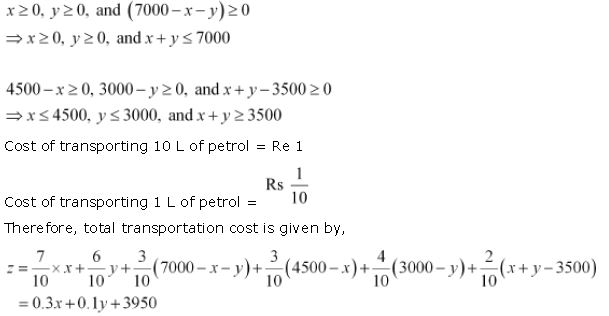The problem can be formulated as follows.

Minimize z = 0.3x + 0.1y + 3950 ...(1)

Subject to the constraints,

x + y ≤ 7000 ...(2)

x ≤ 4500 ...(3)

y ≤ 3000 ...(4)

x + y ≥   3500 ...(5)

x, y ≥ 0 ...(6)

The feasible region determined by the constraints is as follows .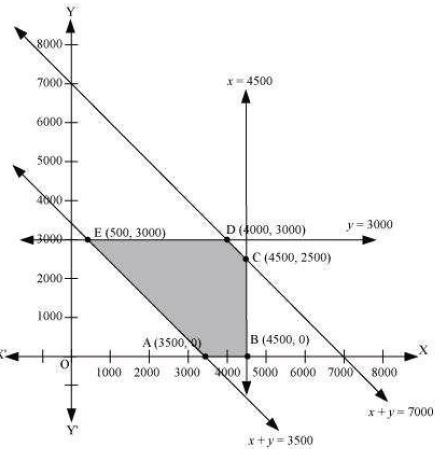The corner points of the feasible region are A (3500, 0), B (4500, 0), C (4500, 2500), D (4000, 3000), and E (500, 3000).

The values of z at these corner points are as follows.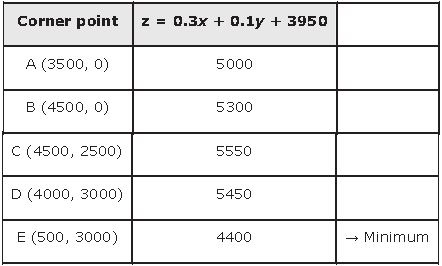The minimum value of z is 4400 at (500, 3000).

Thus, the oil supplied from depot A is 500 L, 3000 L, and 3500 L and from depot B is 4000 L, 0 L, and 0 L to petrol pumps D, E, and F respectively.

The minimum transportation cost is Rs 4400.

Question8. A fruit grower can use two types of fertilizer in his garden, brand P and brand Q. The amounts (in kg) of nitrogen, phosphoric acid, potash, and chlorine in a bag of each brand are given in the table. Tests indicate that the garden needs at least 240 kg of phosphoric acid at least 270 kg of potash and at most 310 kg of chlorine.
If the grower wants to minimize the amount of nitrogen added to the garden, how many bags of each brand should be used? What is the minimum amount of nitrogen added in the garden?Let the fruit grower use x bags of brand P and y bags of brand Q.

The problem can be formulated as follows.

Minimize z = 3x + 3.5y  …(1)

subject to the constraints,

x + 2y ≥ 240  ...(2)

x + 0.5y ≥ 90  ...(3)

1.5x + 2y ≤ 310  ...(4)

x, y ≥ 0  ...(5)

The feasible region determined by the system of constraints is as follows.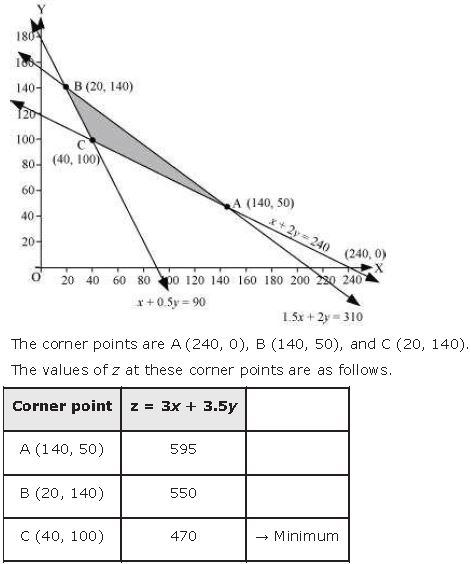The maximum value of z is 470 at (40, 100).

Thus, 40 bags of brand P and 100 bags of brand Q should be added to the garden to minimize the amount of nitrogen.

The minimum amount of nitrogen added to the garden is 470 kg.

Question9. Refer to question 8. If the grower wants to maximize the amount of nitrogen added to the garden, how many bags of each brand should be added? What is the maximum amount of nitrogen added?

Let the fruit grower use x bags of brand P and y bags of brand Q.

The problem can be formulated as follows.

Maximize z = 3x + 3.5y …(1)

subject to the constraints,

x + 2y ≥ 240  ...(2)

x + 0.5y ≥ 90 ...(3)

1.5x + 2y ≤ 310  ...(4)

x, y ≥ 0  ...(5)

The feasible region determined by the system of constraints is as follows.The maximum value of z is 595 at (140, 50).

Thus, 140 bags of brand P and 50 bags of brand Q should be used to maximize the amount of nitrogen.

The maximum amount of nitrogen added to the garden is 595 kg.

Question10. A toy company manufactures two types of dolls, A and B. Market tests and available resources have indicated that the combined production level should not exceed 1200 dolls per week and the demand for dolls of type B is at most half of that for dolls of type A. Further, the production level of dolls of type A can exceed three times the production of dolls of other type by at most 600 units. If the company makes profit of Rs 12 and Rs 16 per doll respectively on dolls A and B, how many of each should be produced weekly in order to maximize the profit?

Let x and y be the number of dolls of type A and B respectively that are produced per week.

The given problem can be formulated as follows.

Maximize z = 12x + 16y …(1)

subject to the constraints,

x + y ≤ 1200 ...(2)

y ≤ x/2 ⇒ x ≥ 2y  ...(3)

x - 3y ≤ 600  ...(4)

x, y ≥ 0  ...(5)

The feasible region determined by the system of constraints is as follows.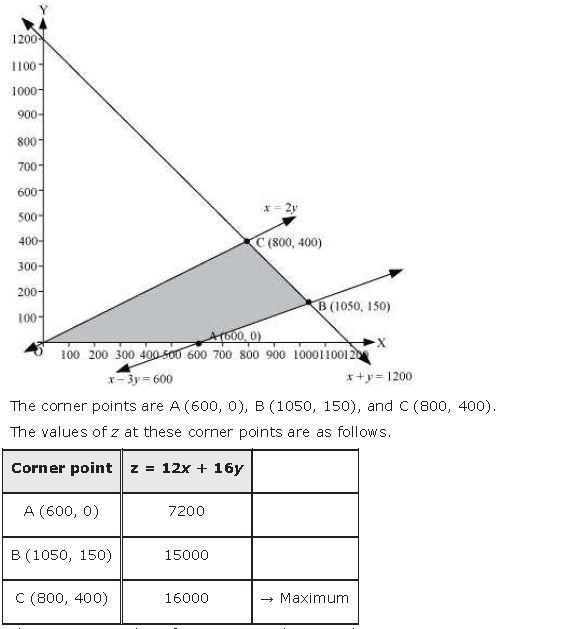The maximum value of z is 16000 at (800, 400).

Thus, 800 and 400 dolls of type A and type B should be produced respectively to get the maximum profit of Rs 16000.

 NCERT Solutions Class 12 Mathematics Chapter 1 Relations and Functions
 NCERT Solutions Class 12 Mathematics Chapter 2 Inverse Trigonometric Functions
 NCERT Solutions Class 12 Mathematics Chapter 3 Matrices
 NCERT Solutions Class 12 Mathematics Chapter 4 Determinants
 NCERT Solutions Class 12 Mathematics Chapter 5 Continuity and Differentiability
 NCERT Solutions Class 12 Mathematics Chapter 6 Application of Derivatives
 NCERT Solutions Class 12 Mathematics Chapter 7 Integrals
 NCERT Solutions Class 12 Mathematics Chapter 8 Application of Integrals
 NCERT Solutions Class 12 Mathematics Chapter 9 Differential Equations
 NCERT Solutions Class 12 Mathematics Chapter 10 Vector Algebra
 NCERT Solutions Class 12 Mathematics Chapter 11 Three Dimensional Geometry
 NCERT Solutions Class 12 Mathematics Chapter 12 Linear Programming
 NCERT Solutions Class 12 Mathematics Chapter 13 Probability

## More Study Material

Tags

### Chapter 12 Linear Programming Class 12 Mathematics NCERT Solutions

The Class 12 Mathematics NCERT Solutions Chapter 12 Linear Programming are designed in a way that will help to improve the overall understanding of students. The answers to each question in Chapter 12 Linear Programming of Mathematics Class 12 has been designed based on the latest syllabus released for the current year. We have also provided detailed explanations for all difficult topics in Chapter 12 Linear Programming Class 12 chapter of Mathematics so that it can be easier for students to understand all answers.

#### NCERT Solutions Chapter 12 Linear Programming Class 12 Mathematics

Class 12 Mathematics NCERT Solutions Chapter 12 Linear Programming is a really good source using which the students can get more marks in exams. The same questions will be coming in your Class 12 Mathematics exam. Learn the Chapter 12 Linear Programming questions and answers daily to get a higher score. Chapter 12 Linear Programming of your Mathematics textbook has a lot of questions at the end of chapter to test the students understanding of the concepts taught in the chapter. Students have to solve the questions and refer to the step-by-step solutions provided by Mathematics teachers on studiestoday to get better problem-solving skills.

#### Chapter 12 Linear Programming Class 12 NCERT Solution Mathematics

These solutions of Chapter 12 Linear Programming NCERT Questions given in your textbook for Class 12 Mathematics have been designed to help students understand the difficult topics of Mathematics in an easy manner. These will also help to build a strong foundation in the Mathematics. There is a combination of theoretical and practical questions relating to all chapters in Mathematics to check the overall learning of the students of Class 12.

#### Class 12 NCERT Solution Mathematics Chapter 12 Linear Programming

NCERT Solutions Class 12 Mathematics Chapter 12 Linear Programming detailed answers are given with the objective of helping students compare their answers with the example. NCERT solutions for Class 12 Mathematics provide a strong foundation for every chapter. They ensure a smooth and easy knowledge of Revision notes for Class 12 Mathematics. As suggested by the HRD ministry, they will perform a major role in JEE. Students can easily download these solutions and use them to prepare for upcoming exams and also go through the Question Papers for Class 12 Mathematics to clarify all doubts

Where can I download latest NCERT Solutions for Class 12 Mathematics Chapter 12 Linear Programming

You can download the NCERT Solutions for Class 12 Mathematics Chapter 12 Linear Programming for latest session from StudiesToday.com

Can I download the NCERT Solutions of Class 12 Mathematics Chapter 12 Linear Programming in Pdf

Yes, you can click on the link above and download NCERT Solutions in PDFs for Class 12 for Mathematics Chapter 12 Linear Programming

Are the Class 12 Mathematics Chapter 12 Linear Programming NCERT Solutions available for the latest session

Yes, the NCERT Solutions issued for Class 12 Mathematics Chapter 12 Linear Programming have been made available here for latest academic session

How can I download the Chapter 12 Linear Programming Class 12 Mathematics NCERT Solutions

You can easily access the links above and download the Chapter 12 Linear Programming Class 12 NCERT Solutions Mathematics for each chapter

Is there any charge for the NCERT Solutions for Class 12 Mathematics Chapter 12 Linear Programming

There is no charge for the NCERT Solutions for Class 12 Mathematics Chapter 12 Linear Programming you can download everything free

How can I improve my scores by reading NCERT Solutions in Class 12 Mathematics Chapter 12 Linear Programming

Regular revision of NCERT Solutions given on studiestoday for Class 12 subject Mathematics Chapter 12 Linear Programming can help you to score better marks in exams

Are there any websites that offer free NCERT solutions for Chapter 12 Linear Programming Class 12 Mathematics

Yes, studiestoday.com provides all latest NCERT Chapter 12 Linear Programming Class 12 Mathematics solutions based on the latest books for the current academic session

Can NCERT solutions for Class 12 Mathematics Chapter 12 Linear Programming be accessed on mobile devices

Yes, studiestoday provides NCERT solutions for Chapter 12 Linear Programming Class 12 Mathematics in mobile-friendly format and can be accessed on smartphones and tablets.

Are NCERT solutions for Class 12 Chapter 12 Linear Programming Mathematics available in multiple languages

Yes, NCERT solutions for Class 12 Chapter 12 Linear Programming Mathematics are available in multiple languages, including English, Hindi